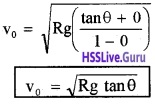# Plus One Physics Notes Chapter 5 Law of Motion

Students can Download Chapter 5 Law of Motion Notes, Plus One Physics Notes helps you to revise the complete Kerala State Syllabus and score more marks in your examinations.

## Kerala Plus One Physics Notes Chapter 5 Law of Motion

Summary
Introduction
In this chapter we are going to learn about the laws that governs the motion of bodies.
Inertia:
The inability of a body to change by itself it’s state of rest or uniform motion along a straight line is called inertia.
Examples of inertia:
1. When a fast moving bus is suddenly stopped, a standing passenger tends to fall in the forward direction.
Explanation
The passenger has the same velocity as that of the bus. When the bus stops suddenly the lower part of his body is brought to rest suddenly because of the friction between his feet and floor of the bus. But the upper part continues to move because of its inertia.2. When a bus suddenly takes off, a standing passenger tends to fall in the backward direction. This is because the lower part of the body gets a speed when the bus picks up speed and upper part continues to be at rest because of its inertia.

3. Consider a person sitting inside a stationary train and tossing a coin. The coin falls into his own hand. If he repeats the experiment when the train is moving with uniform speed, then also the coin falls into his own hand.

4. Cleaning a carpet by beating is in accordance with law of inertia.

5. Rabbit chased by a dog runs in zigzag manner. This is to take advantage of the large inertia of the massive dog.

6. A person chased by an elephant runs in a zigzag manner or in a circle. This is to take the advantage of the large inertia of the massive elephant.

Newton’s Laws:
Newton built on Galileo’s ideas and laid the foundation of mechanics in terms of three laws.

• Newtons first law
• Newtons second law
• Newtons third law

Newton’s First Law Of Motion
Everybody continues in its state of rest or of uniform motion along a straight line unless it is compelled by an external unbalanced force to change that state:
Note: Newton’s first law of motion brings the idea of inertia. Inertia of a body is measured by the mass of the body. Heavier the body, greater is the force required to change its state and hence greater is its inertia.Newton’s Second Law Of Motion
Linear Momentum ($$\vec{p}$$):
Momentum of a body is defined as the product of its mass m and velocity $$\vec{v}$$Explanation
Momentum of a body can be produced or destroyed by the application of force on it. Therefore, momentum of a body is measured by the force required to stop the body in unit time.
Force required to stop a moving body depends upon

1. mass of the body
2. velocity of the body.

1. Mass of the body:
When a ball and a big stone are allowed to fall from the same height, we find that a greater force is required to stop the big piece of stone than the ball. Thus larger the mass of a body, greater is its linear momentum.

2. Velocity of the body:
A bullet thrown with the hand can be stopped easily than the same bullet fired from the gun. Therefore, langerthe velocity of a body, greater is its linear momentum.
Note: Momentum is a vector quantity. Its unit is Kgms-1
Newton’s Second Law of motion:
The rate of change of momentum of a body is directly proportional to the applied force and takes place in the direction in which the force acts. Mathematically this can be written as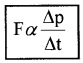Question 1.
Derive F = ma from Newton’s Second law.
Consider a body of mass ‘m’ moving with a momentum $$\vec{p}$$. Let $$\vec{F}$$ be the force acting on it for time internal Dt. Due to this force the momentum is changed from $$\vec{p}$$ to p + Dp. Then according to Newtons second law, we can writeWhere K is a constant proportionality. When we take the limit ∆t → 0, we can writeUnit of force:
Unit of force is newton. 1N = 1Kgms-2
Force in terms of the components:
We know force is a vector, Hence we can write as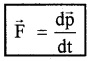Impulsive force:
The forces which act on bodies for short time are called impulsive forces.
Example:

• In hitting a ball with a bat
• In firing a gun

Impulse:
An impulse force does not remain constant, but changes from zero to maximum. This impulsive force is not easy to measure, because it changes with time. In such a case, we measure the total effect of the force called impulse.

The impulse of a force is the product of the average force and the time for which it acts.Relation between impulse and momentum:
We know from Newtons second law
F = $$\frac{\Delta p}{\Delta t}$$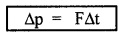R.H.S. is the impulse and L.H.S. is change of momentum ie; change of momentum = impulse.

Question 2.
F = $$\frac{\Delta p}{\Delta t}$$. When we jump to hard soil, Dt is small and F is large. When we jump to loose soil it takes more time for the body to come to rest. Therefore, Dt is large and F will be small.

Question 3.
A cricketer draws his hand while catching a cricket ball. Why?
When cricketer draws his hand, the Dt will increase. Hence F acting on the hand will decrease.Newtons Third Law Of Motion
Statement:
To every action, there is always an equal and opposite reaction.
Explanation: When a book is placed on the table, the weight of the book acts on the table downwards. The table exerts an equal force on the book in the upward direction. If the force applied by the book on the table is action, the force applied by the table on the book is reaction.

Question 4.
If action and reaction are equal and opposite, why they do not cancel?
Though action and reaction are equal and opposite, they do not cancel each other because action is on one body and reaction is on another body.
Consider a pair of bodies A and B. According to the
third law FAB = – FBA
(force on A by B) = – (force on B by A).

Conservation Of Momentum
Second law and third law lead to conservation of linear momentum.
Statement:
When there is no external force on a body (or system), the total momentum remains constant.

Proof in the case of a single body:
According to Newtons second law, F = $$\frac{d p}{d t}$$. if F = 0, we get p = constant. Which means that momentum of a body remains constant, if there is no external force acting on it.

Conservation of momentum in the case of firing a gun:
Consider a gun of mass M and bullet of mass ‘m’ at rest. On firing the gun exerts a force F on the bullet and bullet exerts an equal force -F in the opposite direction. Because of this action and reaction (due to firing), the gun acquires a momentum Pg and bullet acquires a momentum Pb.
Momentum before firing
The bullet and gun are at rest. Hence momentum before firing = M × 0 + m × 0
Momentum before firing = 0 ________(1)
Momentum after firing
According to Newtons second law, the change in
momentum of bullet. ∆Pb = Pb – 0 = F∆t ______(2)
Since initially both are rest,
Dp = final momentum – initial
momentum Similarly the change in momentum of gun
∆pg = pg – 0 = -F∆t _______(3)
∴ Total momentum after firing = pb + pg
= F∆t + – F∆t.
Total momentum after firing = 0 _______(4)
from eq (1) and eq (4), we get,
Total momentum before firing = Total momentum after firing.Conservation of momentum in the case of two colliding bodies: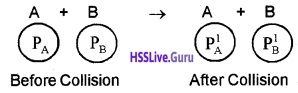Consider two bodies A and B with initial momenta PA and PB. After collision, they acquire momenta P1A and P1g respectively.
According to Newton’s second law, the change in momentum of A due to the collision with B,Similarly the change in momentum of B due to the collision with A, FBA∆t = P1B – PB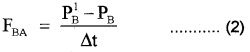[Where Dt is time for which the two bodies are in contact].
According Newton’s third law, we can write
FAB = -FBATotal momentum before collision = Total momentum after collision.
Note: Conservation linear momentum is always satisfied for elastic collision and inelastic collision.

Equilibrium Of A Particle
Equilibrium of a particle in mechanics refers to the situation, when the net external force on the particle is zero.

Common Forces In Mechanics
There are two types of forces commonly used in mechanics,

1. Contact forces
2. Non contact forces

1. Contact forces:
A contact force on an object arises due to contact with some other object. Example : Friction, viscosity, air resistance etc.

2. Non contact forces:
A non contact force on an object arises due to non contact with some other object
Example: Gravitational force

Friction:
Friction is the force that develops at the surfaces of contact of two bodies and impedes (opposes) their relative motion.
There are different types of friction.• Static friction: The opposing force that comes into play when one body tends to move over the surface of another (but the actual motion has yet not started)
• Limiting friction (fs): The maximum value of static friction is called limiting friction.
• Kinetic friction (fk)(or) dynamic friction: Kinetic friction or dynamic friction is the opposing force that comes into play when one body is actually moving’overthe surface of another body.
• Sliding friction: The opposing force that comes into play when one body is actually sliding over the surface of the other body is called sliding friction.
• Rolling friction: The opposing force that comes into play when one body is actually rolling over the surface of the other body is called rolling friction.

Laws of static Friction:

• The force of maximum static friction is directly proportional to the normal reaction
• The force of static friction is opposite to the direction in which the body tends to move.
• The force of static friction is parallel to the surfaces in contact.
• The force of maximum static friction is independent of the area of contact (as long as the normal reaction remains constant).
• The force of static friction depends only on the nature of surfaces in contact.a. Laws of Kinetic friction:

1. The force of Kinetic friction is proportional to normal reaction.
2. The force of Kinetic friction is opposite to the dh rection in which the body moves.
3. The force of Kinetic friction is parallel to the surfaces in contact.
4. The force of Kinetic friction is independent of the area contact (as long as the normal reaction remains constant)
5. The force of Kinetic friction depends on the nature of surface.
6. Force of Kinetic friction is almost independent of the speed.
7. Force of Kinetic friction is less than force of static friction.

b. Coefficient of static friction:
The force of static friction (fs)max is directly proportional to the normal reaction N
(fs)max α N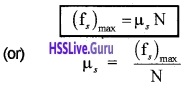Where ms is called coefficient of static friction.
Definition of ms
Coefficient of static friction is the ratio of the force of the maximum static friction to the nprmal reaction.

c. Coefficient of Kinetic friction:
The force of kinetic friction is directly proportional to the normal reaction N.
i e (fk)max α NWhere µk is called coefficient of Kinetic friction.
Definition of µk
Coefficient of Kinetic friction is the ratio of the force of Kinetic friction to the normal reaction.

d. Angle of friction:
Angle of friction is the angle whose tangent gives the coefficient of friction.Proof:
Consider a body placed on a surface. Let N be the normal reaction and flimit is the limiting friction. Let ‘θ’ be the angle between Resultant vector and normal reaction. From the triangle OBC,∴ tanθ = µ
Angle of repose:
The angle of repose is the angle of the inclined plane at which a body placed of it just begins to slide.
Explanation
considers body placed on a inclined plane. Gradually increase the angle of inclination till the body placed on its surface just begins to slide down. If α is the inclination at which the body just begins to slide down, then α is called angle of repose.The limiting friction F acts in upward direction along the inclined plane. When the body just begins to move, we can write
F = mg sin α ______(1)
from the figure normal reaction,
N = mg cos α ______(2)
dividing eq (1) by eq (2)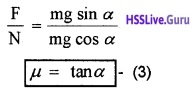Note: Angle of repose is equal to angle of friction.
Rolling friction:
Why rolling friction is less than kinetic friction?
When a body rolls over a plane, there is just one point of contact between the body and plane. The relative motion between point and plane is zero. Hence in this ideal situation, kinetic friction becomes zero.

• Friction helps us to walk on the ground.
• Friction helps us to hold objects.
• Friction helps in striking matches.
• Friction helps in driving automobiles.
• Friction is helpful in stopping a vehicle etc.

• Friction produces wear and tear.
• Friction leads to wastage of energy in the form of heat.
• Friction reduces the efficiency of the engine etc.

Steps to reduce friction

• Polishing the surfaces in contact
• Use of lubricants
• Ball bearing placed between moving parts of machine.Circular Motion
When a body moves along circumstances of a circle, there is an acceleration towards it’s centre. This acceleration is called centripetal acceleration. The force providing this acceleration is called centripetal force.
Centripetal force f = $$\frac{\mathrm{mv}^{2}}{\mathrm{R}}$$

1. For a stone rotated in a circle by a string, the centripetal force is provided by the tension in the string.
2. The centripetal force for motion of a planet around the sun is the gravitational force on the planet due to sun.
3. For a car on circular road, the centripetal force is provided by the friction between tire and road.

1. Motion of a car on a level road: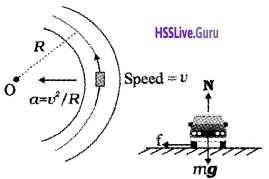Consider a vehicle moving overa level curved road. The two forces acting on it are

• Weight (mg) vertically down
• The reaction (N)

The normal reaction can’t produce sufficient centripetal force required for circular motion. The centripetal force for circular motion is provided by friction. This friction opposes the motion of the car moving away from the circular road. Hence condition for circular motion can be written as Centripetal force ≤ force of frictionThe maximum speed of circular motion of the car
vmax = $$\sqrt{\mu_{s} \mathrm{rg}}$$

Question 5.
Why surface of the road is kept inclined to the horizontal?
Consider a vehicle moving along a level curved road. The vehicle will have a tendency to slip outward. This outward slip is prevented by frictional force. But friction causes unnecessary wear and tear. More over, for typical value of µ and R the maximum speed v = $$\sqrt{\mu_{s} \mathrm{rg}}$$ rg will be very small.

These defects can be avoided if we raise the outer edge of the road slightly above the inner edge. This process is called banking of curve. The angle made by the surface of the road with the horizontal is called the angle of banking.

2. Motion of a car on a banked road: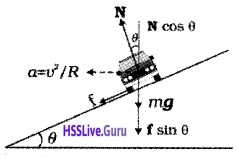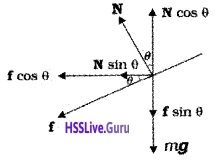Consider a vehicle along a curved road with angle of banking q. Then the normal reaction on the ground will be inclined at an angle q with the vertical.The vertical component can be divided into N Cosq (vertical component) and N sinq (horizontal component). Suppose the vehicle has a tendency to slip outward. Then the frictional force will be developed along the plane of road as shown in the figure. The frictional force can be divided into two components. Fcosq (horizontal component) and F sinq (vertical component).
From the figure are get
N cos q = F sinq + mg
N cosq – F sinq = mg ______(1)
The component Nsinq and Fsinq provide centripetal force. HenceDividing both numerator and denominator of L.H.S by N cosq. We get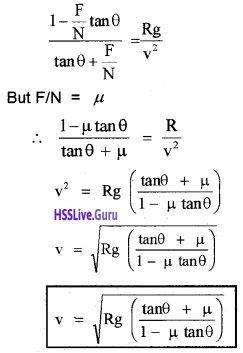This is the maximum speed at which vehicle can move over a banked curved road.Optimum speed:
Optimum speed is the speed at which a vehicle can move over a curved banked road without using unnecessary friction.
When a car is moved with optimum speed Vo, m can be taken as zero.
putting m = 0 in the above equation we get